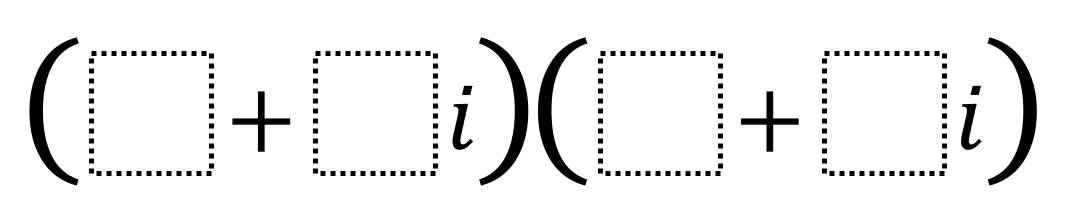# Multiplying Complex Numbers 2

Directions: Using the integers -9 to 9 at most one time each, fill in the boxes to make a real number product with the greatest possible value.### Hint

What is the difference between a real and complex number? What causes an i to go away during multiplication? What causes a term to go away during binomial multiplication? How do we choose integers such that the i terms are additive inverses of one another?

There are two possible answers: (-9 + 6i)(-6 + -4i) and (9 + -6i)(6 + 4i)

Source: Robert Kaplinsky in Open Middle Math

## The Modulus Of A Complex Number

Directions: Using the digits 0 to 9 at most one time each, place a digit …

### One comment

1.How can we prove that this is the biggest product possible?